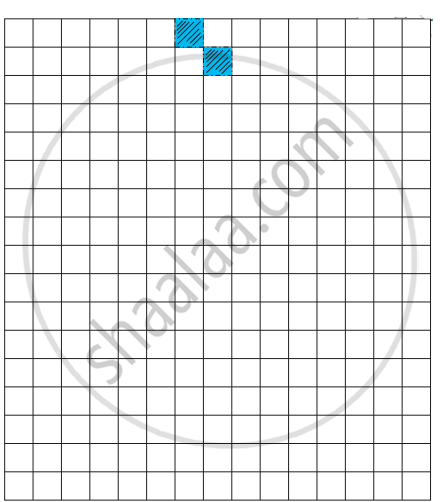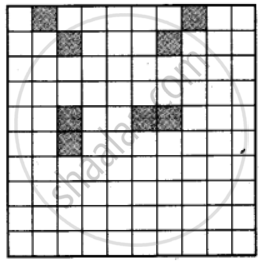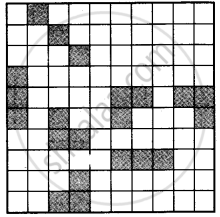# How many different shapes can you make by joining two squares? Draw them on the squared sheet given below. How long is the boundary of each shape? - Mathematics

1. How many different shapes can you make by joining two squares? Draw them on the squared sheet given below. How long is the boundary of each shape?2. Try this activity with three squares also.

#### Solution

1. The shapes made by joining two squares are shown here :There are four types of shapes that can be made by joining two squares.
2. Following types of shapes can be made by joining three squares.Seven types of shapes can be made by joining three squares.

Concept: Fields and Fences
Is there an error in this question or solution?

#### APPEARS IN

NCERT Mathematics - Math - Magic Class 4 CBSE
Chapter 13 Fields and Fences
Exercises | Q 3. | Page 154